Скачать презентацию l l l

2662a328fe184c705a1dd6f696ce44db.ppt

• Количество слайдов: 48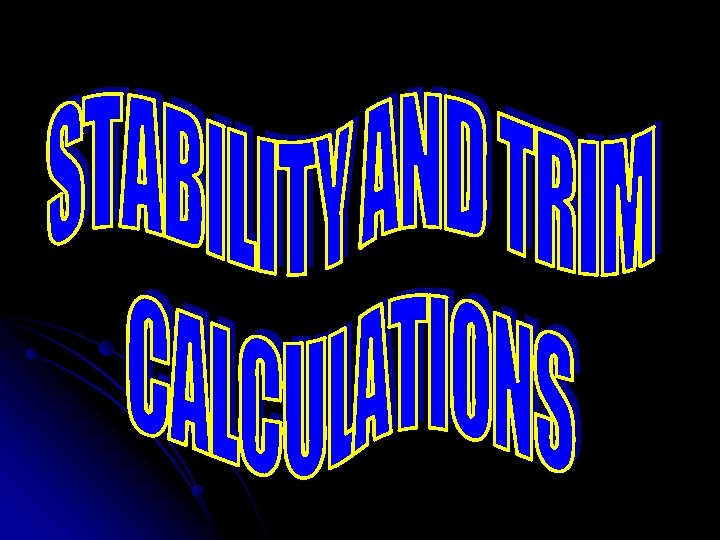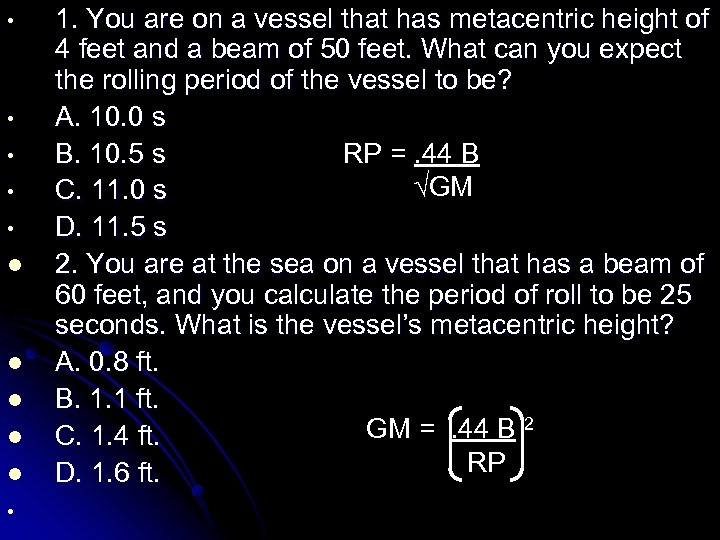• • • l l l • 1. You are on a vessel that has metacentric height of 4 feet and a beam of 50 feet. What can you expect the rolling period of the vessel to be? A. 10. 0 s B. 10. 5 s RP =. 44 B √GM C. 11. 0 s D. 11. 5 s 2. You are at the sea on a vessel that has a beam of 60 feet, and you calculate the period of roll to be 25 seconds. What is the vessel’s metacentric height? A. 0. 8 ft. B. 1. 1 ft. GM =. 44 B 2 C. 1. 4 ft. RP D. 1. 6 ft.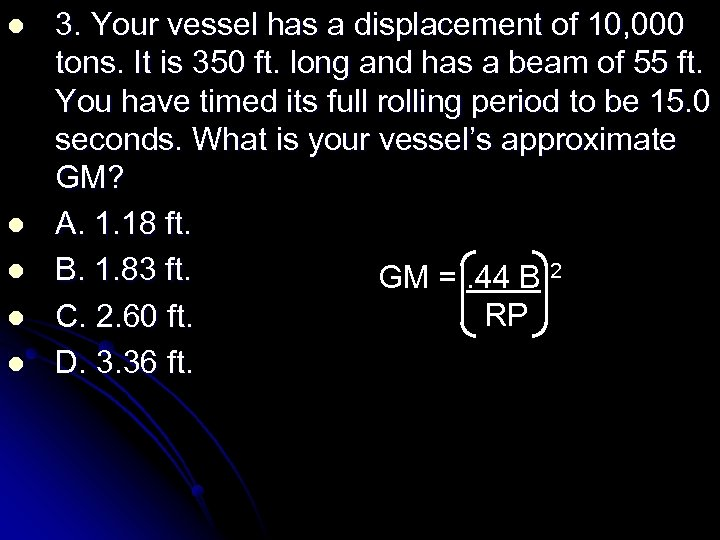l l l 3. Your vessel has a displacement of 10, 000 tons. It is 350 ft. long and has a beam of 55 ft. You have timed its full rolling period to be 15. 0 seconds. What is your vessel’s approximate GM? A. 1. 18 ft. B. 1. 83 ft. GM =. 44 B 2 RP C. 2. 60 ft. D. 3. 36 ft.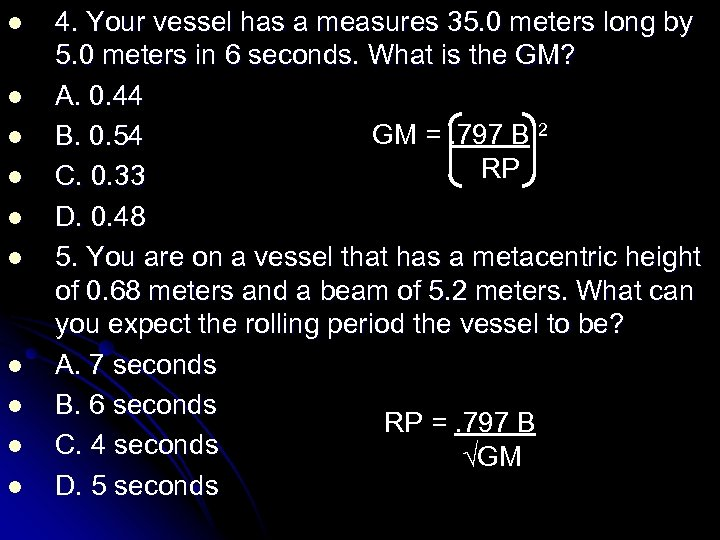l l l l l 4. Your vessel has a measures 35. 0 meters long by 5. 0 meters in 6 seconds. What is the GM? A. 0. 44 GM =. 797 B 2 B. 0. 54 RP C. 0. 33 D. 0. 48 5. You are on a vessel that has a metacentric height of 0. 68 meters and a beam of 5. 2 meters. What can you expect the rolling period the vessel to be? A. 7 seconds B. 6 seconds RP =. 797 B C. 4 seconds √GM D. 5 seconds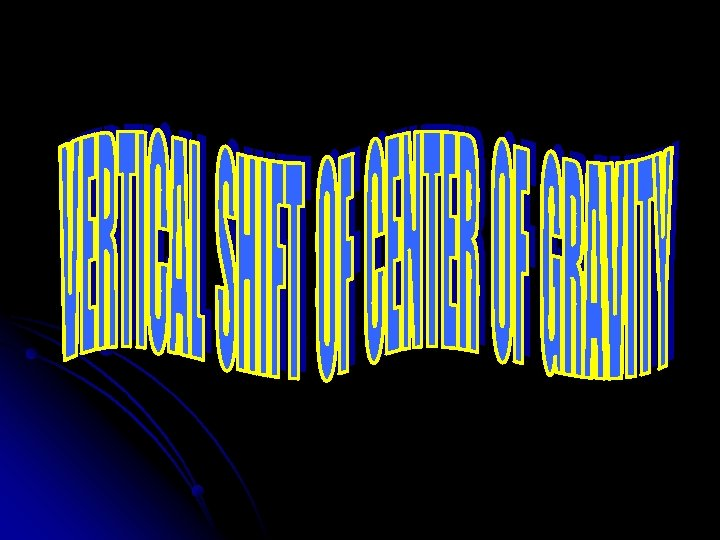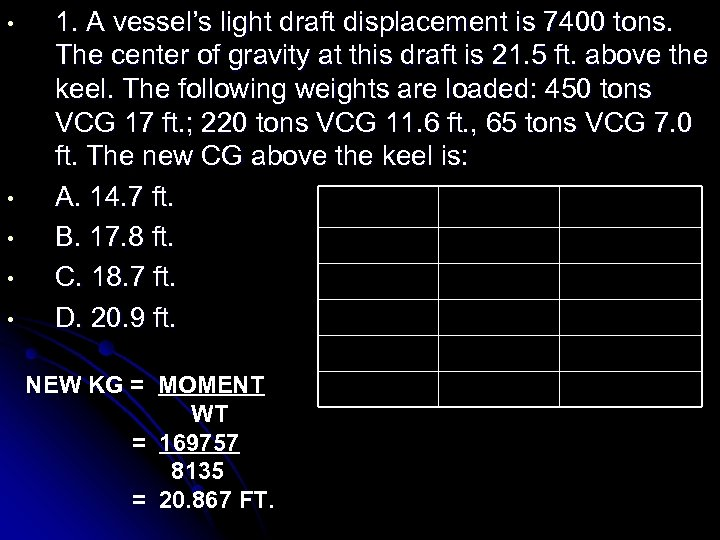• • • 1. A vessel’s light draft displacement is 7400 tons. The center of gravity at this draft is 21. 5 ft. above the keel. The following weights are loaded: 450 tons VCG 17 ft. ; 220 tons VCG 11. 6 ft. , 65 tons VCG 7. 0 ft. The new CG above the keel is: A. 14. 7 ft. WEIGHT C. G MOMENT B. 17. 8 ft. 7400 21. 5 159100 C. 18. 7 ft. (+) 450 17. 0 7650 (+) 220 11. 6 2552 D. 20. 9 ft. NEW KG = MOMENT WT = 169757 8135 = 20. 867 FT. (+) 65 8135 7. 0 455 169757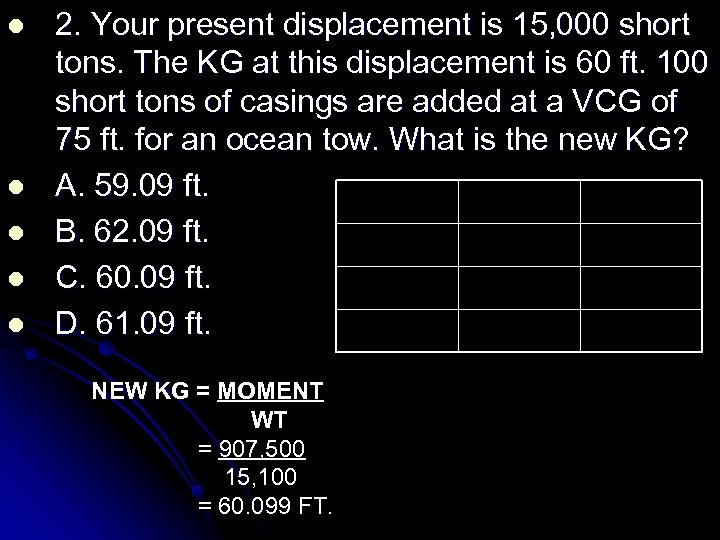l l l 2. Your present displacement is 15, 000 short tons. The KG at this displacement is 60 ft. 100 short tons of casings are added at a VCG of 75 ft. for an ocean tow. What is the new KG? A. 59. 09 ft. WEIGHT KG MOMENT B. 62. 09 ft. 15, 000 60 900, 000 C. 60. 09 ft. (+) 100 75 (+) 7, 500 D. 61. 09 ft. 15, 100 907, 500 NEW KG = MOMENT WT = 907, 500 15, 100 = 60. 099 FT.l l l 3. A vessel with displacement of 19, 700 LT and a KG of 50. 96 ft. loads 300 LT of barite into P-tanks located 120 ft. above the keel. What is the change in KG? WEIGHT KG MOMENT A. 1. 00 ft. upward 19, 700 50. 96 1, 003, 912 B. 0. 79 ft. upward (+) 300 120 (+)36, 000 C. 1. 04 ft. upward 20, 000 1, 039, 912 D. 1. 83 ft. upward NEW KG = MOMENT WT = 1, 039, 912 20, 000 = 52. 00 OLD KG = 50. 96 (-) 1. 04 UPWARD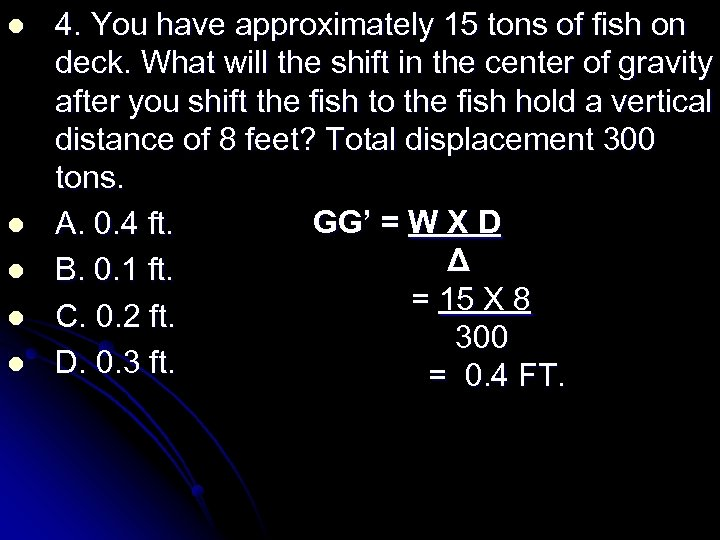l l l 4. You have approximately 15 tons of fish on deck. What will the shift in the center of gravity after you shift the fish to the fish hold a vertical distance of 8 feet? Total displacement 300 tons. GG’ = W X D A. 0. 4 ft. Δ B. 0. 1 ft. = 15 X 8 C. 0. 2 ft. 300 D. 0. 3 ft. = 0. 4 FT.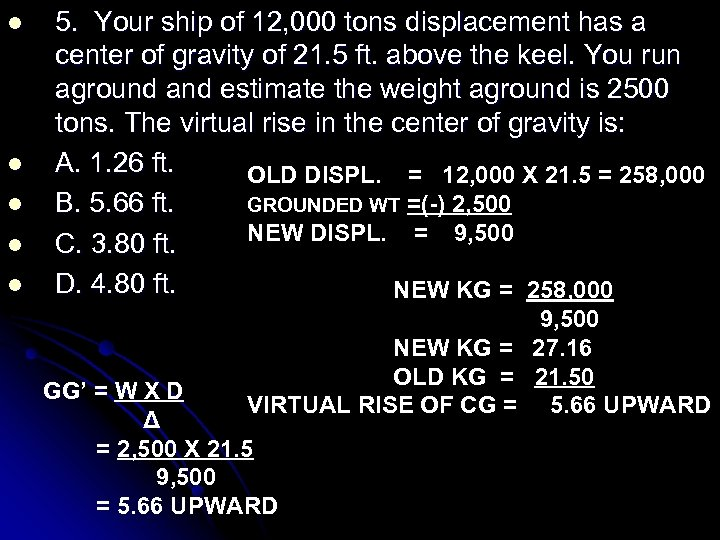l l l 5. Your ship of 12, 000 tons displacement has a center of gravity of 21. 5 ft. above the keel. You run aground and estimate the weight aground is 2500 tons. The virtual rise in the center of gravity is: A. 1. 26 ft. OLD DISPL. = 12, 000 X 21. 5 = 258, 000 GROUNDED WT =(-) 2, 500 B. 5. 66 ft. NEW DISPL. = 9, 500 C. 3. 80 ft. D. 4. 80 ft. NEW KG = 258, 000 9, 500 NEW KG = 27. 16 OLD KG = 21. 50 VIRTUAL RISE OF CG = 5. 66 UPWARD GG’ = W X D Δ = 2, 500 X 21. 5 9, 500 = 5. 66 UPWARD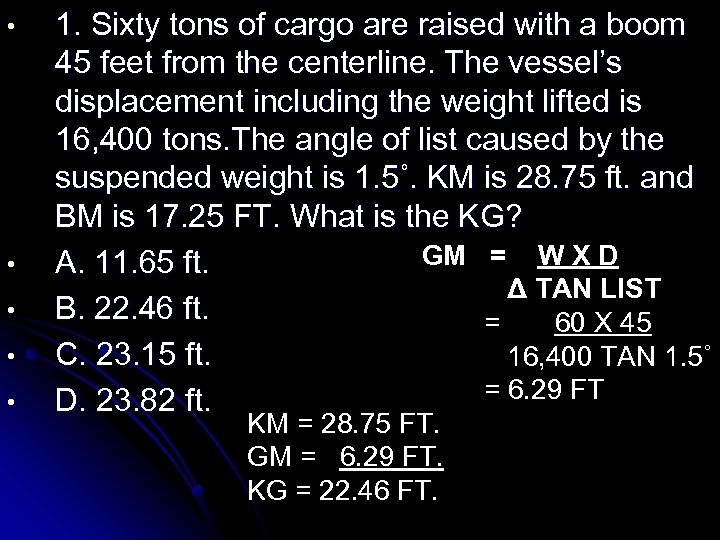• • • 1. Sixty tons of cargo are raised with a boom 45 feet from the centerline. The vessel’s displacement including the weight lifted is 16, 400 tons. The angle of list caused by the suspended weight is 1. 5˚. KM is 28. 75 ft. and BM is 17. 25 FT. What is the KG? GM = W X D A. 11. 65 ft. Δ TAN LIST B. 22. 46 ft. = 60 X 45 C. 23. 15 ft. 16, 400 TAN 1. 5˚ = 6. 29 FT D. 23. 82 ft. KM = 28. 75 FT. GM = 6. 29 FT. KG = 22. 46 FT.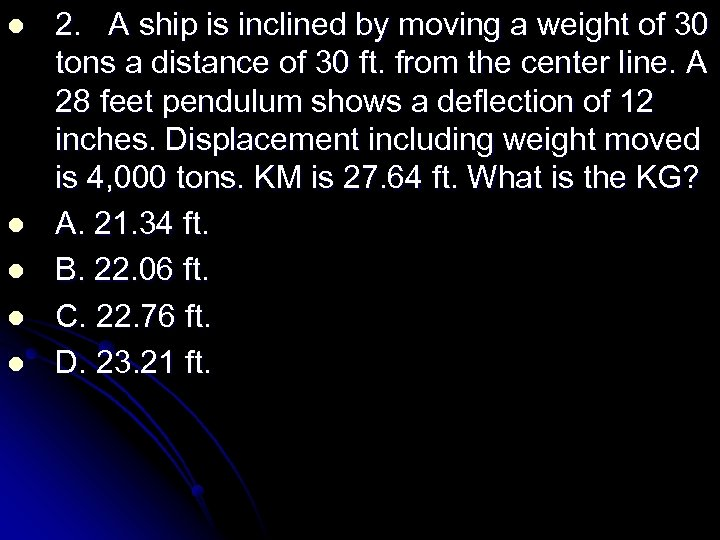l l l 2. A ship is inclined by moving a weight of 30 tons a distance of 30 ft. from the center line. A 28 feet pendulum shows a deflection of 12 inches. Displacement including weight moved is 4, 000 tons. KM is 27. 64 ft. What is the KG? A. 21. 34 ft. B. 22. 06 ft. C. 22. 76 ft. D. 23. 21 ft.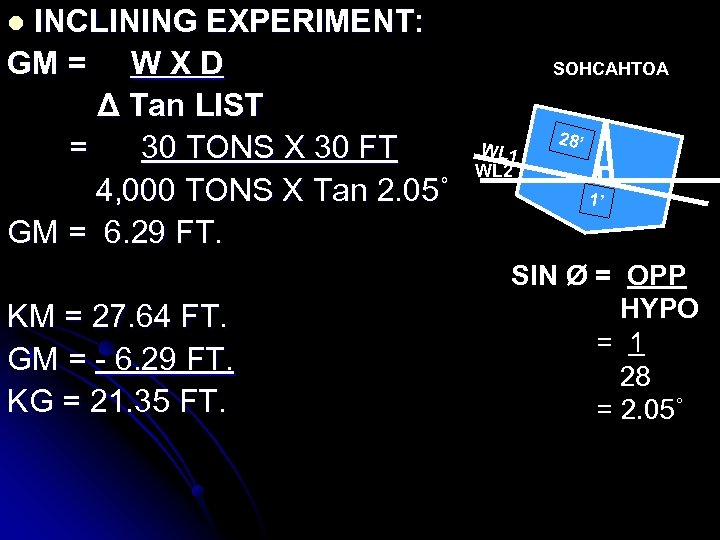INCLINING EXPERIMENT: GM = W X D Δ Tan LIST = 30 TONS X 30 FT 4, 000 TONS X Tan 2. 05˚ GM = 6. 29 FT. l KM = 27. 64 FT. GM = - 6. 29 FT. KG = 21. 35 FT. SOHCAHTOA WL 1 WL 2 28’ 1’ SIN Ø = OPP HYPO = 1 28 = 2. 05˚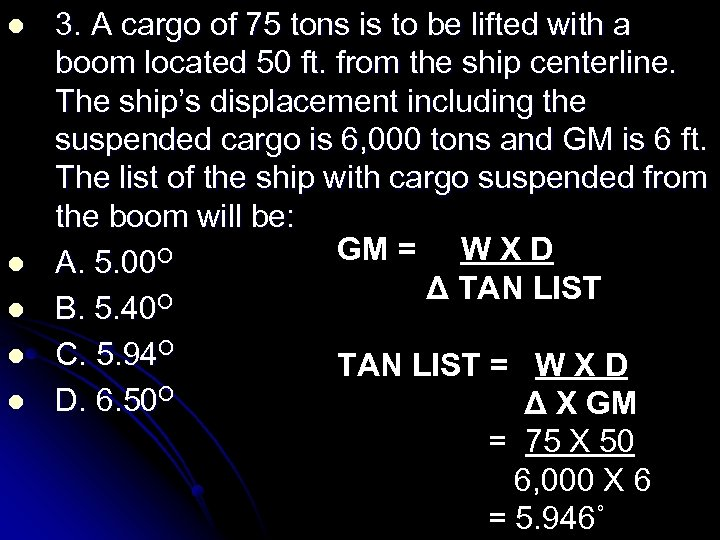l l l 3. A cargo of 75 tons is to be lifted with a boom located 50 ft. from the ship centerline. The ship’s displacement including the suspended cargo is 6, 000 tons and GM is 6 ft. The list of the ship with cargo suspended from the boom will be: GM = W X D A. 5. 00 O Δ TAN LIST O B. 5. 40 C. 5. 94 O TAN LIST = W X D D. 6. 50 O Δ X GM = 75 X 50 6, 000 X 6 = 5. 946˚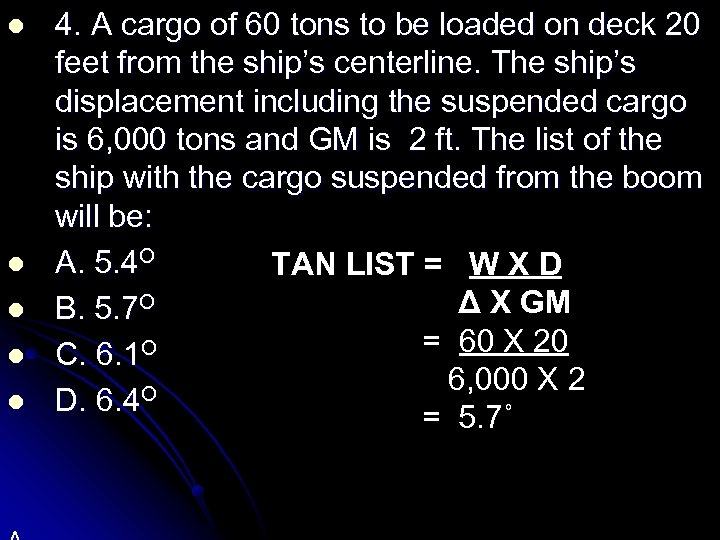l l l 4. A cargo of 60 tons to be loaded on deck 20 feet from the ship’s centerline. The ship’s displacement including the suspended cargo is 6, 000 tons and GM is 2 ft. The list of the ship with the cargo suspended from the boom will be: A. 5. 4 O TAN LIST = W X D Δ X GM B. 5. 7 O = 60 X 20 O C. 6. 1 6, 000 X 2 O D. 6. 4 = 5. 7˚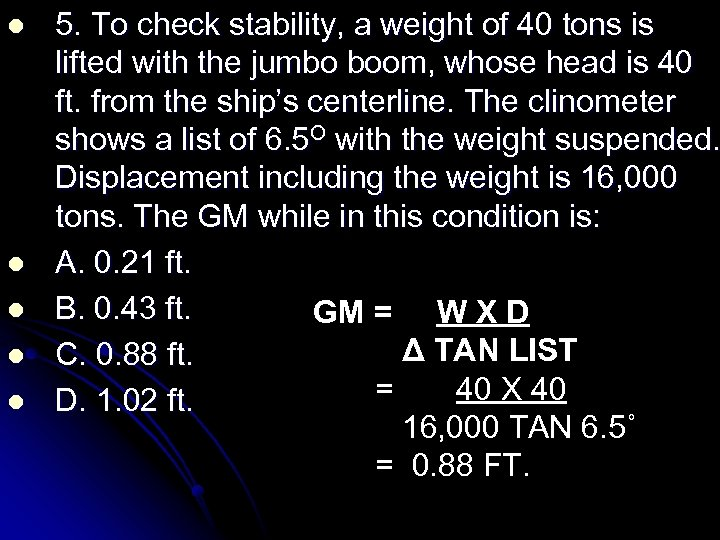l l l 5. To check stability, a weight of 40 tons is lifted with the jumbo boom, whose head is 40 ft. from the ship’s centerline. The clinometer shows a list of 6. 5 O with the weight suspended. Displacement including the weight is 16, 000 tons. The GM while in this condition is: A. 0. 21 ft. B. 0. 43 ft. GM = W X D Δ TAN LIST C. 0. 88 ft. = 40 X 40 D. 1. 02 ft. 16, 000 TAN 6. 5˚ = 0. 88 FT.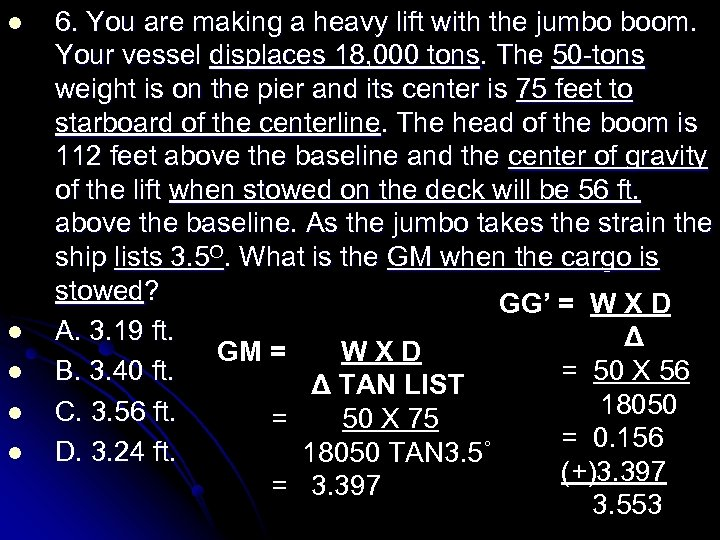l l l 6. You are making a heavy lift with the jumbo boom. Your vessel displaces 18, 000 tons. The 50 -tons weight is on the pier and its center is 75 feet to starboard of the centerline. The head of the boom is 112 feet above the baseline and the center of gravity of the lift when stowed on the deck will be 56 ft. above the baseline. As the jumbo takes the strain the ship lists 3. 5 O. What is the GM when the cargo is stowed? GG’ = W X D A. 3. 19 ft. Δ GM = WXD = 50 X 56 B. 3. 40 ft. Δ TAN LIST 18050 C. 3. 56 ft. = 50 X 75 = 0. 156 D. 3. 24 ft. 18050 TAN 3. 5˚ (+)3. 397 = 3. 397 3. 553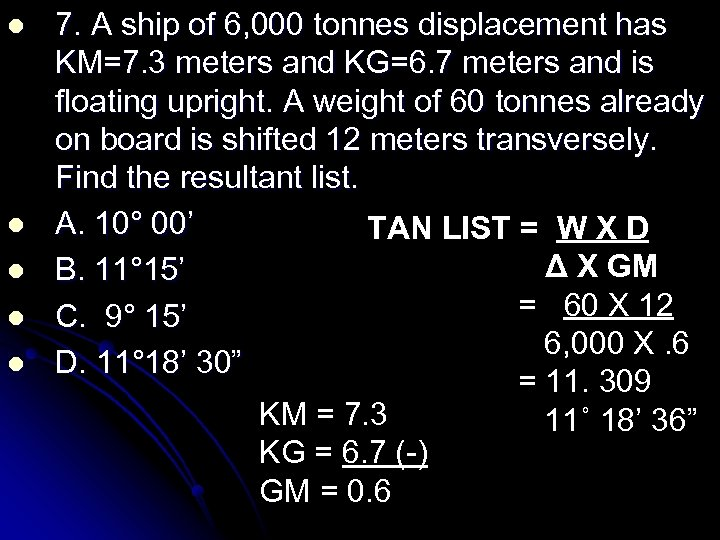l l l 7. A ship of 6, 000 tonnes displacement has KM=7. 3 meters and KG=6. 7 meters and is floating upright. A weight of 60 tonnes already on board is shifted 12 meters transversely. Find the resultant list. A. 10° 00’ TAN LIST = W X D Δ X GM B. 11° 15’ = 60 X 12 C. 9° 15’ 6, 000 X. 6 D. 11° 18’ 30” = 11. 309 KM = 7. 3 11˚ 18’ 36” KG = 6. 7 (-) GM = 0. 6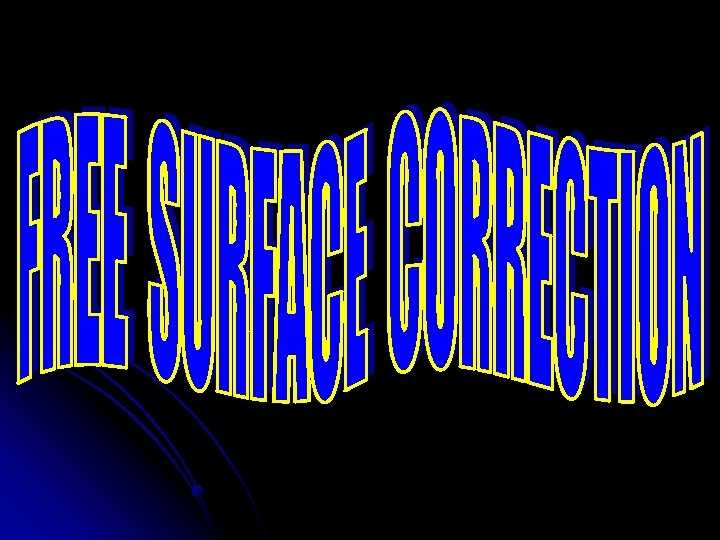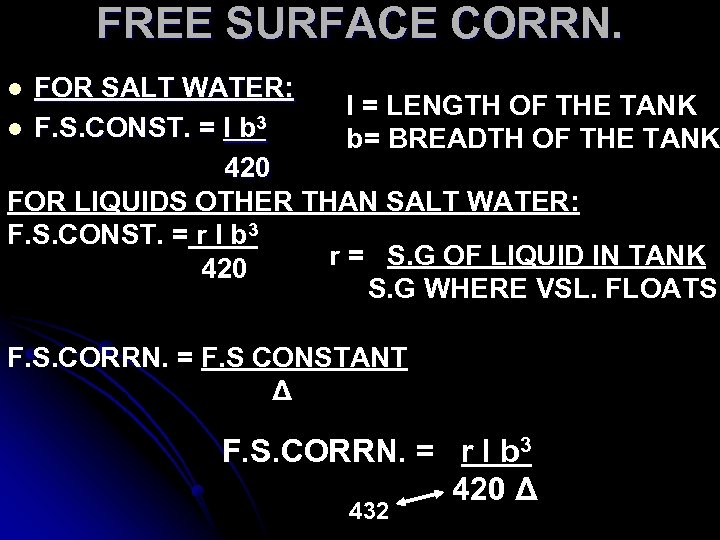FREE SURFACE CORRN. FOR SALT WATER: l = LENGTH OF THE TANK l F. S. CONST. = l b 3 b= BREADTH OF THE TANK 420 FOR LIQUIDS OTHER THAN SALT WATER: F. S. CONST. = r l b 3 r = S. G OF LIQUID IN TANK 420 S. G WHERE VSL. FLOATS l F. S. CORRN. = F. S CONSTANT Δ F. S. CORRN. = r l b 3 420 Δ 432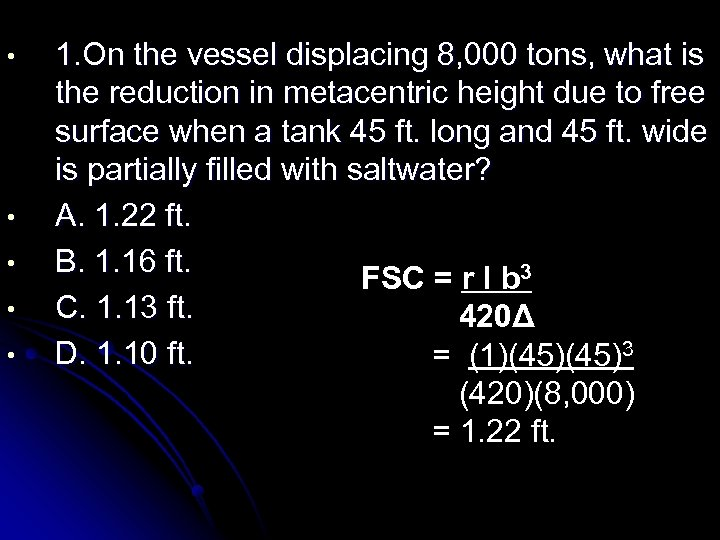• • • 1. On the vessel displacing 8, 000 tons, what is the reduction in metacentric height due to free surface when a tank 45 ft. long and 45 ft. wide is partially filled with saltwater? A. 1. 22 ft. B. 1. 16 ft. FSC = r l b 3 C. 1. 13 ft. 420Δ D. 1. 10 ft. = (1)(45)3 (420)(8, 000) = 1. 22 ft.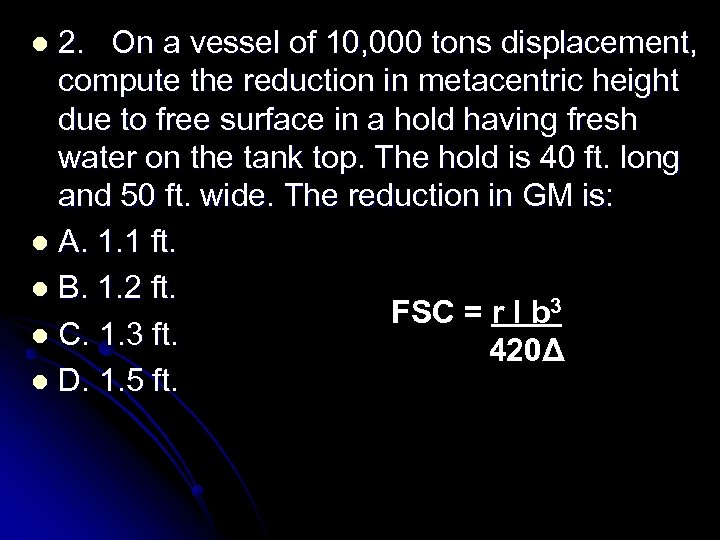2. On a vessel of 10, 000 tons displacement, compute the reduction in metacentric height due to free surface in a hold having fresh water on the tank top. The hold is 40 ft. long and 50 ft. wide. The reduction in GM is: l A. 1. 1 ft. l B. 1. 2 ft. FSC = r l b 3 l C. 1. 3 ft. 420Δ l D. 1. 5 ft. l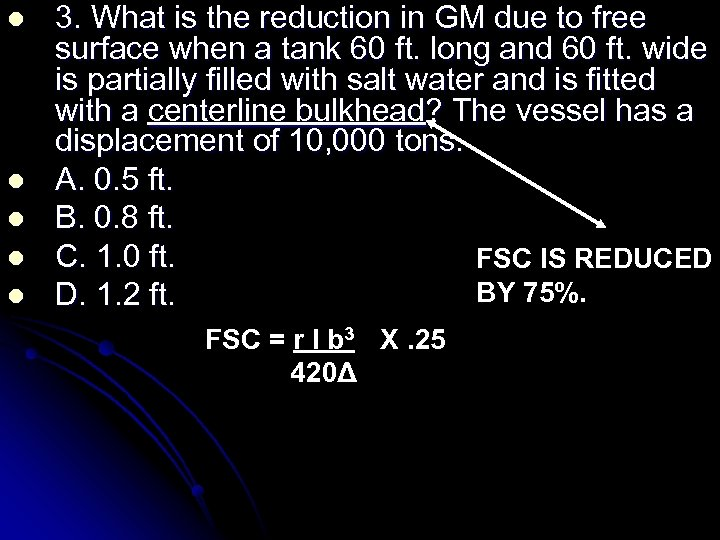l l l 3. What is the reduction in GM due to free surface when a tank 60 ft. long and 60 ft. wide is partially filled with salt water and is fitted with a centerline bulkhead? The vessel has a displacement of 10, 000 tons. A. 0. 5 ft. B. 0. 8 ft. C. 1. 0 ft. FSC IS REDUCED BY 75%. D. 1. 2 ft. FSC = r l b 3 X. 25 420Δ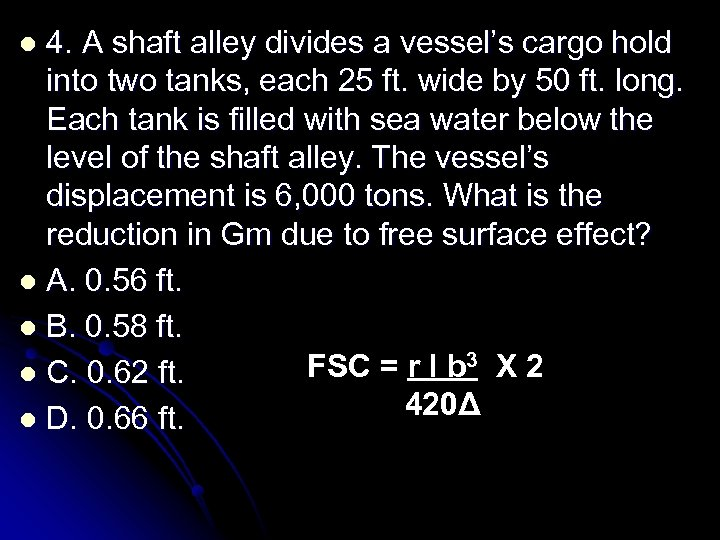4. A shaft alley divides a vessel’s cargo hold into two tanks, each 25 ft. wide by 50 ft. long. Each tank is filled with sea water below the level of the shaft alley. The vessel’s displacement is 6, 000 tons. What is the reduction in Gm due to free surface effect? l A. 0. 56 ft. l B. 0. 58 ft. FSC = r l b 3 X 2 l C. 0. 62 ft. 420Δ l D. 0. 66 ft. l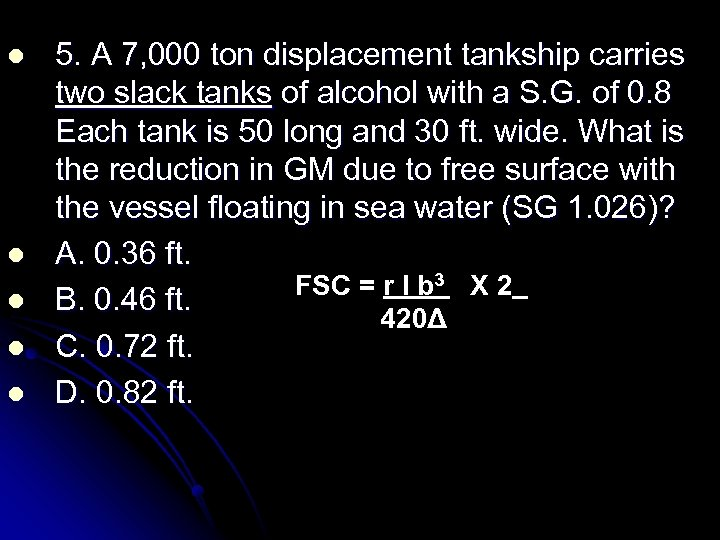l l l 5. A 7, 000 ton displacement tankship carries two slack tanks of alcohol with a S. G. of 0. 8 Each tank is 50 long and 30 ft. wide. What is the reduction in GM due to free surface with the vessel floating in sea water (SG 1. 026)? A. 0. 36 ft. FSC = r l b 3 X 2 B. 0. 46 ft. 420Δ C. 0. 72 ft. D. 0. 82 ft.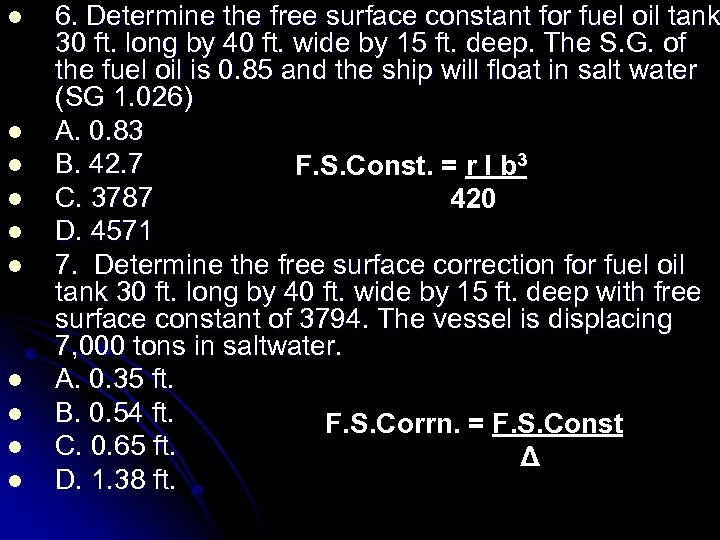l l l l l 6. Determine the free surface constant for fuel oil tank 30 ft. long by 40 ft. wide by 15 ft. deep. The S. G. of the fuel oil is 0. 85 and the ship will float in salt water (SG 1. 026) A. 0. 83 B. 42. 7 F. S. Const. = r l b 3 C. 3787 420 D. 4571 7. Determine the free surface correction for fuel oil tank 30 ft. long by 40 ft. wide by 15 ft. deep with free surface constant of 3794. The vessel is displacing 7, 000 tons in saltwater. A. 0. 35 ft. B. 0. 54 ft. F. S. Corrn. = F. S. Const C. 0. 65 ft. Δ D. 1. 38 ft.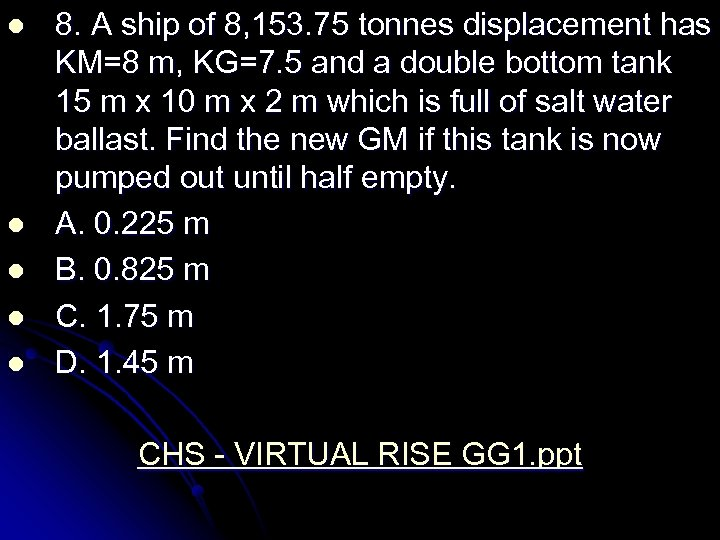l l l 8. A ship of 8, 153. 75 tonnes displacement has KM=8 m, KG=7. 5 and a double bottom tank 15 m x 10 m x 2 m which is full of salt water ballast. Find the new GM if this tank is now pumped out until half empty. A. 0. 225 m B. 0. 825 m C. 1. 75 m D. 1. 45 m CHS - VIRTUAL RISE GG 1. ppt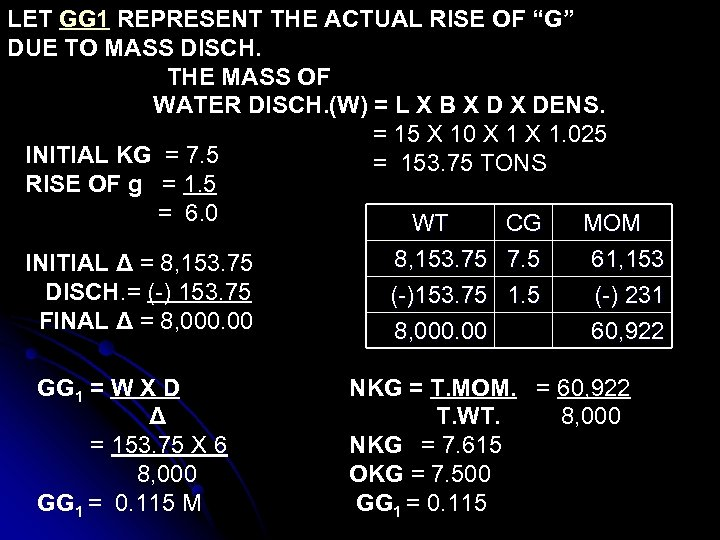LET GG 1 REPRESENT THE ACTUAL RISE OF “G” DUE TO MASS DISCH. THE MASS OF WATER DISCH. (W) = L X B X DENS. = 15 X 10 X 1. 025 INITIAL KG = 7. 5 = 153. 75 TONS RISE OF g = 1. 5 = 6. 0 WT CG MOM INITIAL Δ = 8, 153. 75 DISCH. = (-) 153. 75 FINAL Δ = 8, 000. 00 GG 1 = W X D Δ = 153. 75 X 6 8, 000 GG 1 = 0. 115 M 8, 153. 75 7. 5 (-)153. 75 1. 5 8, 000. 00 61, 153 (-) 231 60, 922 NKG = T. MOM. = 60, 922 T. WT. 8, 000 NKG = 7. 615 OKG = 7. 500 GG 1 = 0. 115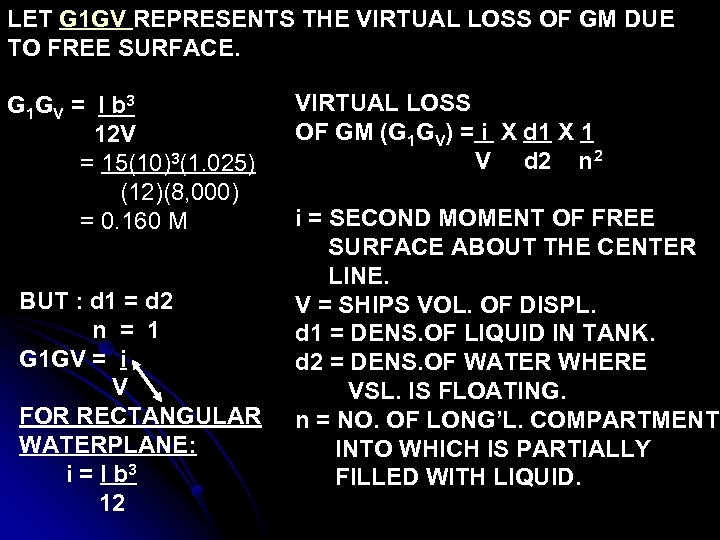LET G 1 GV REPRESENTS THE VIRTUAL LOSS OF GM DUE TO FREE SURFACE. G 1 G V = l b 3 12 V = 15(10)3(1. 025) (12)(8, 000) = 0. 160 M BUT : d 1 = d 2 n = 1 G 1 GV = i V FOR RECTANGULAR WATERPLANE: i = l b 3 12 VIRTUAL LOSS OF GM (G 1 GV) = i X d 1 X 1 V d 2 n 2 i = SECOND MOMENT OF FREE SURFACE ABOUT THE CENTER LINE. V = SHIPS VOL. OF DISPL. d 1 = DENS. OF LIQUID IN TANK. d 2 = DENS. OF WATER WHERE VSL. IS FLOATING. n = NO. OF LONG’L. COMPARTMENT INTO WHICH IS PARTIALLY FILLED WITH LIQUID.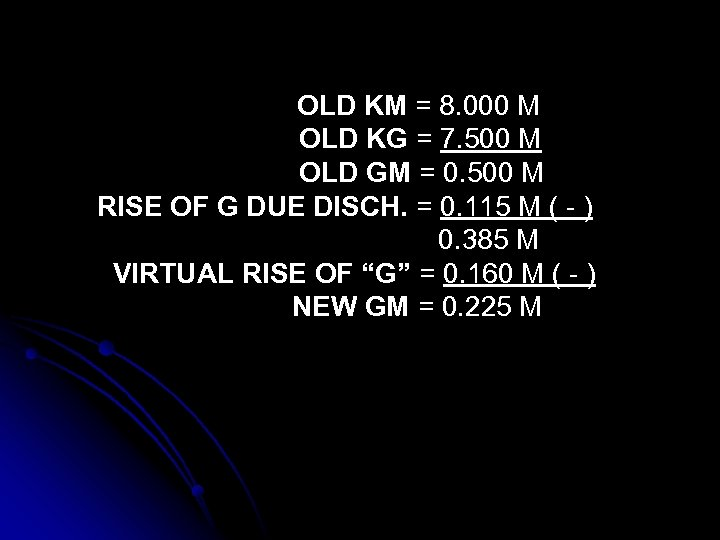OLD KM = 8. 000 M OLD KG = 7. 500 M OLD GM = 0. 500 M RISE OF G DUE DISCH. = 0. 115 M ( - ) 0. 385 M VIRTUAL RISE OF “G” = 0. 160 M ( - ) NEW GM = 0. 225 M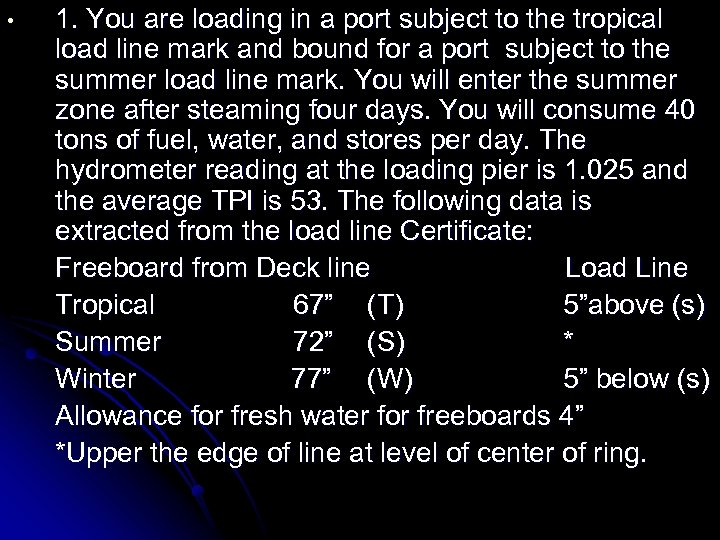• 1. You are loading in a port subject to the tropical load line mark and bound for a port subject to the summer load line mark. You will enter the summer zone after steaming four days. You will consume 40 tons of fuel, water, and stores per day. The hydrometer reading at the loading pier is 1. 025 and the average TPI is 53. The following data is extracted from the load line Certificate: Freeboard from Deck line Load Line Tropical 67” (T) 5”above (s) Summer 72” (S) * Winter 77” (W) 5” below (s) Allowance for fresh water for freeboards 4” *Upper the edge of line at level of center of ring.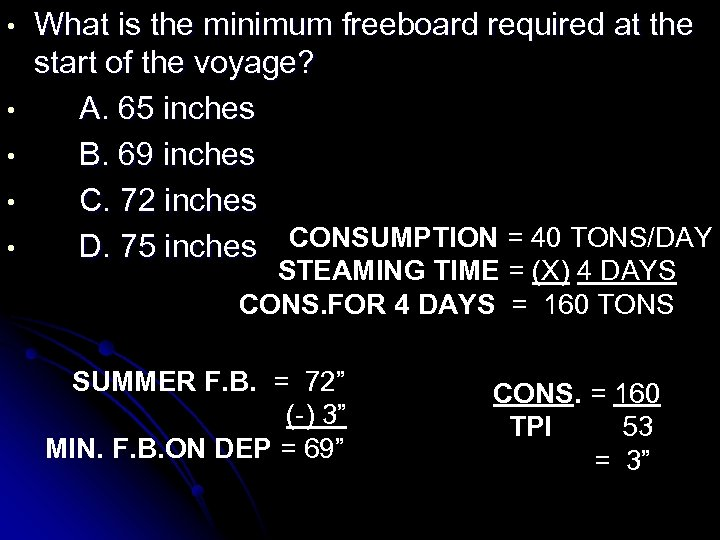• • • What is the minimum freeboard required at the start of the voyage? A. 65 inches B. 69 inches C. 72 inches D. 75 inches CONSUMPTION = 40 TONS/DAY STEAMING TIME = (X) 4 DAYS CONS. FOR 4 DAYS = 160 TONS SUMMER F. B. = 72” (-) 3” MIN. F. B. ON DEP = 69” CONS. = 160 TPI 53 = 3”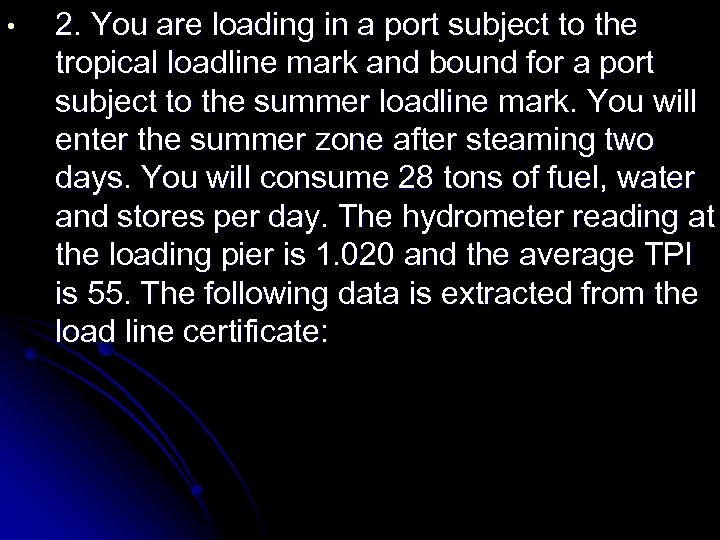• 2. You are loading in a port subject to the tropical loadline mark and bound for a port subject to the summer loadline mark. You will enter the summer zone after steaming two days. You will consume 28 tons of fuel, water and stores per day. The hydrometer reading at the loading pier is 1. 020 and the average TPI is 55. The following data is extracted from the load line certificate: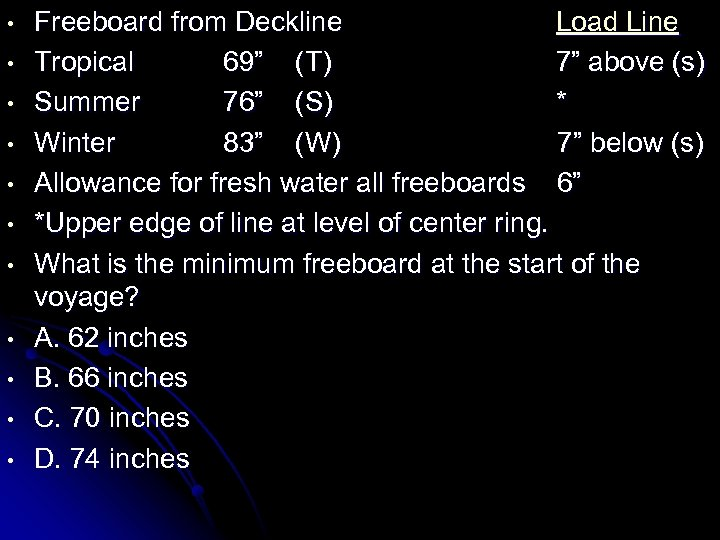• • • Freeboard from Deckline Load Line Tropical 69” (T) 7” above (s) Summer 76” (S) * Winter 83” (W) 7” below (s) Allowance for fresh water all freeboards 6” *Upper edge of line at level of center ring. What is the minimum freeboard at the start of the voyage? A. 62 inches B. 66 inches C. 70 inches D. 74 inches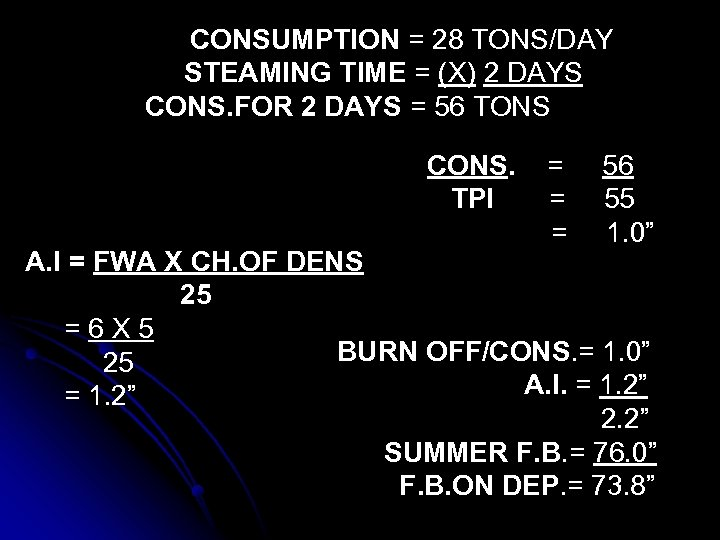CONSUMPTION = 28 TONS/DAY STEAMING TIME = (X) 2 DAYS CONS. FOR 2 DAYS = 56 TONS CONS. TPI = = = 56 55 1. 0” A. I = FWA X CH. OF DENS 25 =6 X 5 BURN OFF/CONS. = 1. 0” 25 A. I. = 1. 2” 2. 2” SUMMER F. B. = 76. 0” F. B. ON DEP. = 73. 8”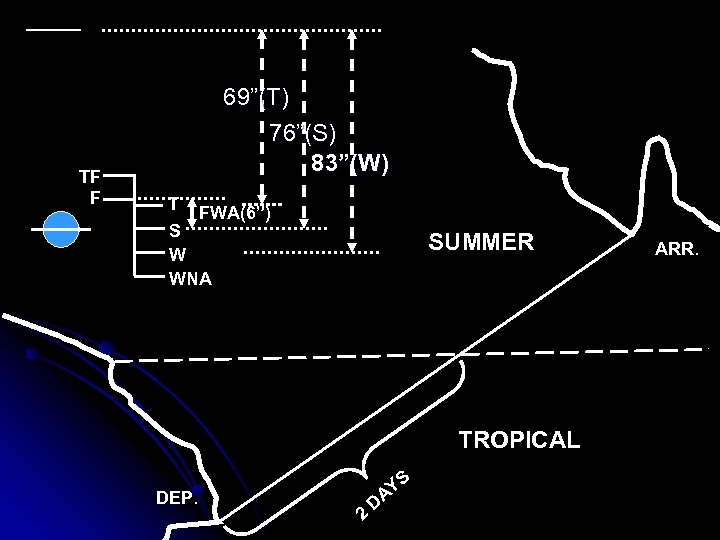TF F 69”(T) 76”(S) 83”(W) T FWA(6”) S W WNA SUMMER TROPICAL DEP. 2 D YS A ARR.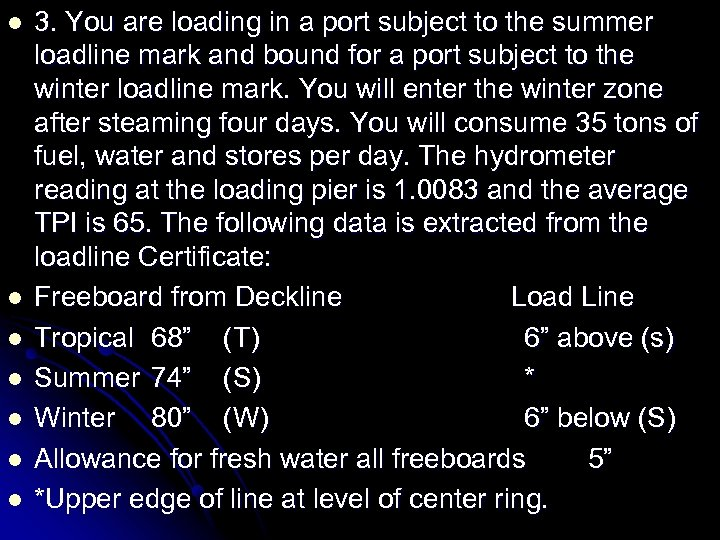l l l l 3. You are loading in a port subject to the summer loadline mark and bound for a port subject to the winter loadline mark. You will enter the winter zone after steaming four days. You will consume 35 tons of fuel, water and stores per day. The hydrometer reading at the loading pier is 1. 0083 and the average TPI is 65. The following data is extracted from the loadline Certificate: Freeboard from Deckline Load Line Tropical 68” (T) 6” above (s) Summer 74” (S) * Winter 80” (W) 6” below (S) Allowance for fresh water all freeboards 5” *Upper edge of line at level of center ring.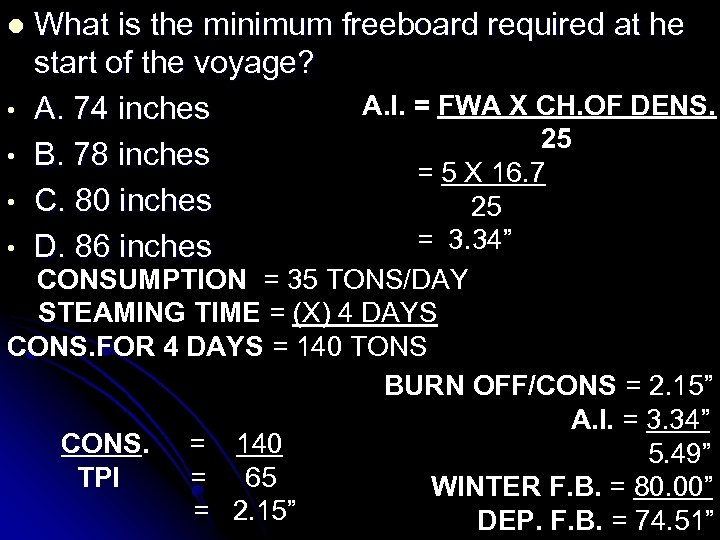l • • What is the minimum freeboard required at he start of the voyage? A. I. = FWA X CH. OF DENS. A. 74 inches 25 B. 78 inches = 5 X 16. 7 C. 80 inches 25 = 3. 34” D. 86 inches CONSUMPTION = 35 TONS/DAY STEAMING TIME = (X) 4 DAYS CONS. FOR 4 DAYS = 140 TONS BURN OFF/CONS = 2. 15” A. I. = 3. 34” CONS. = 140 5. 49” TPI = 65 WINTER F. B. = 80. 00” = 2. 15” DEP. F. B. = 74. 51”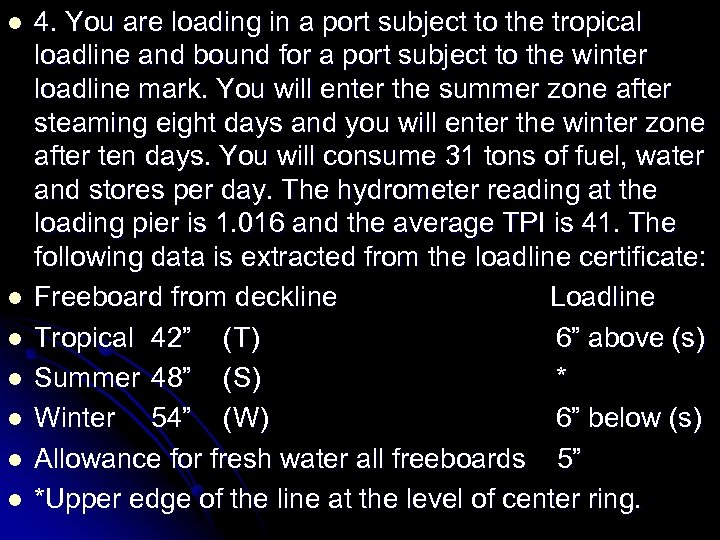l l l l 4. You are loading in a port subject to the tropical loadline and bound for a port subject to the winter loadline mark. You will enter the summer zone after steaming eight days and you will enter the winter zone after ten days. You will consume 31 tons of fuel, water and stores per day. The hydrometer reading at the loading pier is 1. 016 and the average TPI is 41. The following data is extracted from the loadline certificate: Freeboard from deckline Loadline Tropical 42” (T) 6” above (s) Summer 48” (S) * Winter 54” (W) 6” below (s) Allowance for fresh water all freeboards 5” *Upper edge of the line at the level of center ring.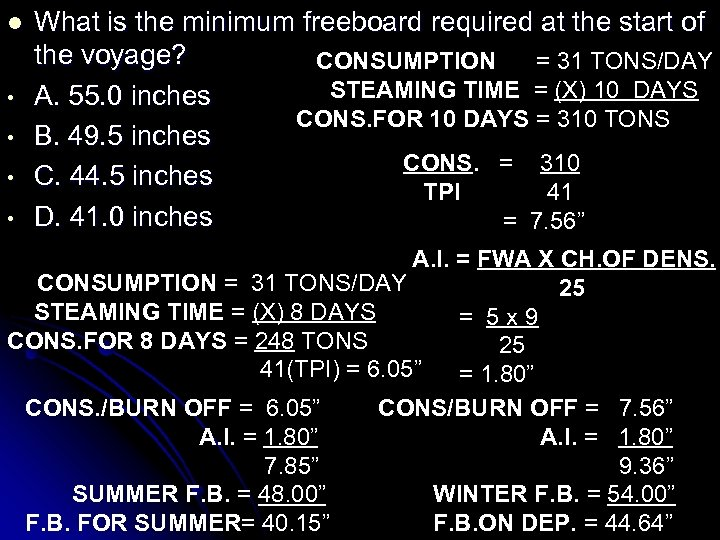l • • What is the minimum freeboard required at the start of the voyage? CONSUMPTION = 31 TONS/DAY STEAMING TIME = (X) 10 DAYS A. 55. 0 inches CONS. FOR 10 DAYS = 310 TONS B. 49. 5 inches CONS. = 310 C. 44. 5 inches TPI 41 D. 41. 0 inches = 7. 56” A. I. = FWA X CH. OF DENS. CONSUMPTION = 31 TONS/DAY 25 STEAMING TIME = (X) 8 DAYS = 5 x 9 CONS. FOR 8 DAYS = 248 TONS 25 41(TPI) = 6. 05” = 1. 80” CONS. /BURN OFF = 6. 05” CONS/BURN OFF = 7. 56” A. I. = 1. 80” 7. 85” 9. 36” SUMMER F. B. = 48. 00” WINTER F. B. = 54. 00” F. B. FOR SUMMER= 40. 15” F. B. ON DEP. = 44. 64”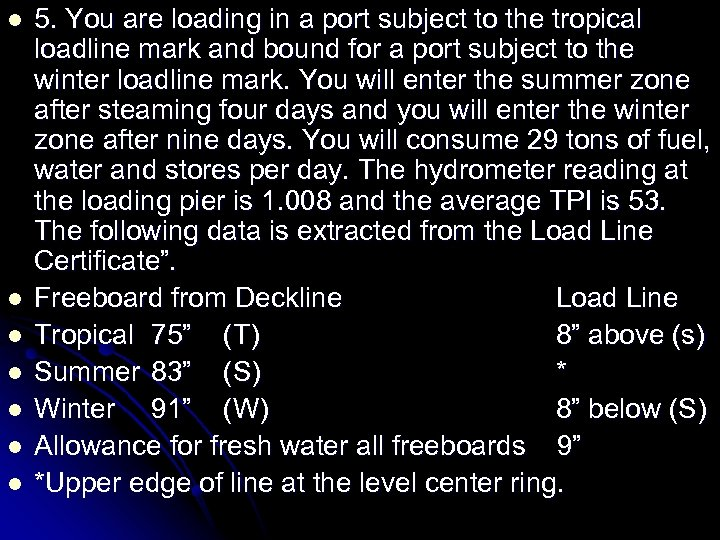l l l l 5. You are loading in a port subject to the tropical loadline mark and bound for a port subject to the winter loadline mark. You will enter the summer zone after steaming four days and you will enter the winter zone after nine days. You will consume 29 tons of fuel, water and stores per day. The hydrometer reading at the loading pier is 1. 008 and the average TPI is 53. The following data is extracted from the Load Line Certificate”. Freeboard from Deckline Load Line Tropical 75” (T) 8” above (s) Summer 83” (S) * Winter 91” (W) 8” below (S) Allowance for fresh water all freeboards 9” *Upper edge of line at the level center ring.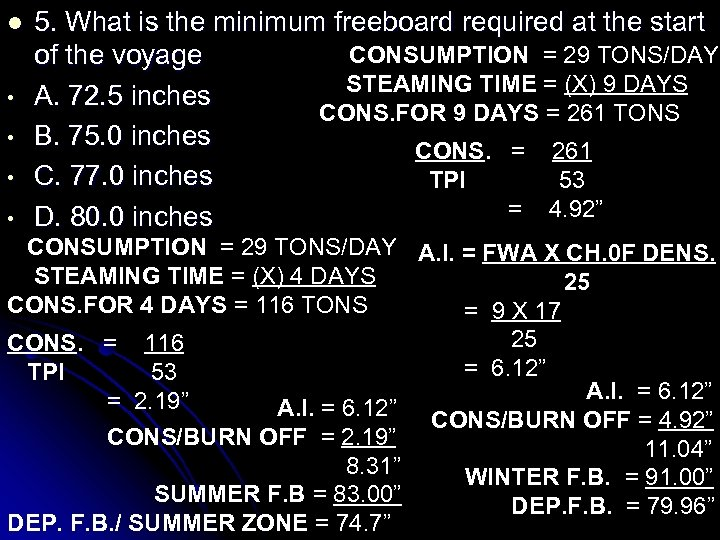l • • 5. What is the minimum freeboard required at the start CONSUMPTION = 29 TONS/DAY of the voyage STEAMING TIME = (X) 9 DAYS A. 72. 5 inches CONS. FOR 9 DAYS = 261 TONS B. 75. 0 inches CONS. = 261 C. 77. 0 inches TPI 53 = 4. 92” D. 80. 0 inches CONSUMPTION = 29 TONS/DAY A. I. = FWA X CH. 0 F DENS. STEAMING TIME = (X) 4 DAYS 25 CONS. FOR 4 DAYS = 116 TONS = 9 X 17 25 CONS. = 116 = 6. 12” TPI 53 A. I. = 6. 12” = 2. 19” A. I. = 6. 12” CONS/BURN OFF = 4. 92” CONS/BURN OFF = 2. 19” 11. 04” 8. 31” WINTER F. B. = 91. 00” SUMMER F. B = 83. 00” DEP. F. B. = 79. 96” DEP. F. B. / SUMMER ZONE = 74. 7”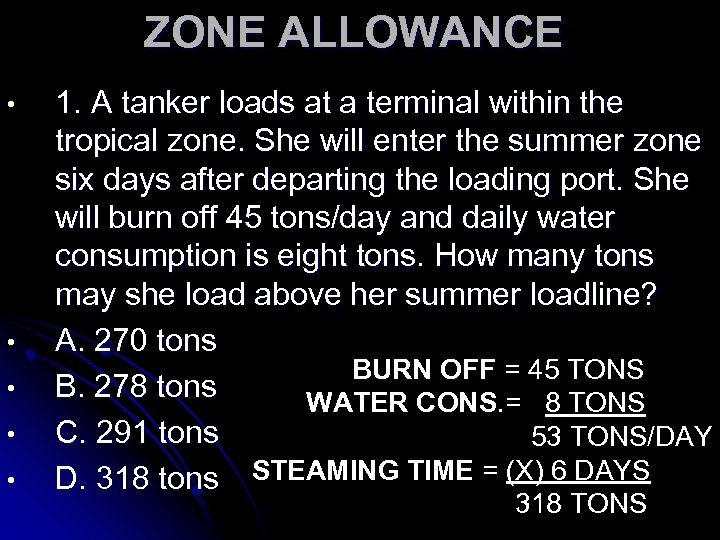ZONE ALLOWANCE • • • 1. A tanker loads at a terminal within the tropical zone. She will enter the summer zone six days after departing the loading port. She will burn off 45 tons/day and daily water consumption is eight tons. How many tons may she load above her summer loadline? A. 270 tons BURN OFF = 45 TONS B. 278 tons WATER CONS. = 8 TONS C. 291 tons 53 TONS/DAY D. 318 tons STEAMING TIME = (X) 6 DAYS 318 TONS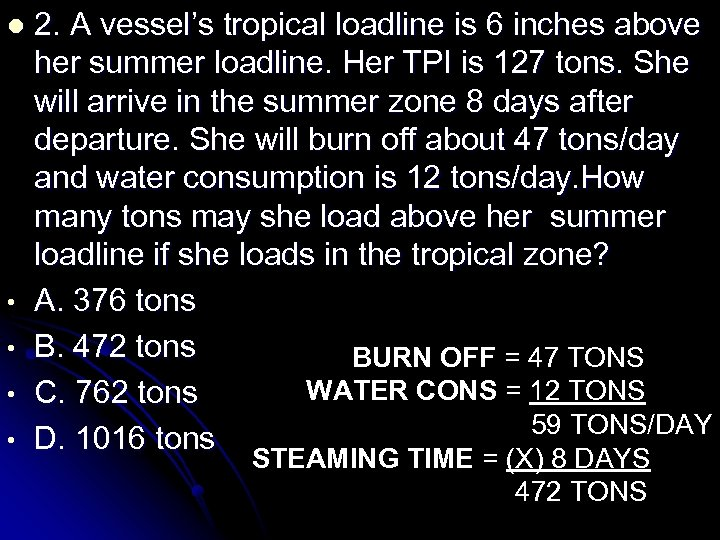l • • 2. A vessel’s tropical loadline is 6 inches above her summer loadline. Her TPI is 127 tons. She will arrive in the summer zone 8 days after departure. She will burn off about 47 tons/day and water consumption is 12 tons/day. How many tons may she load above her summer loadline if she loads in the tropical zone? A. 376 tons B. 472 tons BURN OFF = 47 TONS WATER CONS = 12 TONS C. 762 tons 59 TONS/DAY D. 1016 tons STEAMING TIME = (X) 8 DAYS 472 TONS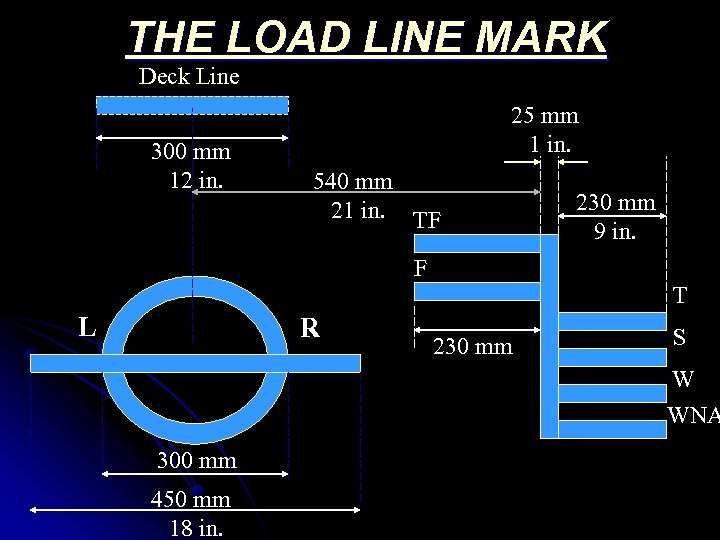THE LOAD LINE MARK Deck Line 300 mm 12 in. 25 mm 1 in. 540 mm 21 in. TF 230 mm 9 in. F T L R 230 mm S W WNA 300 mm 450 mm 18 in.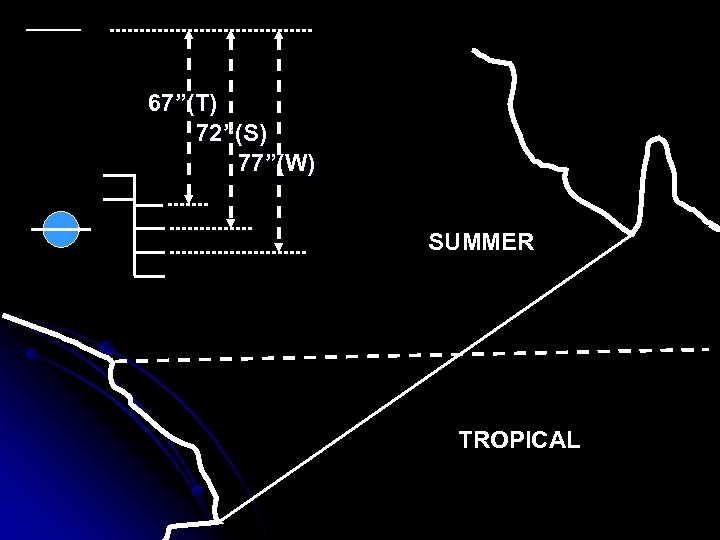67”(T) 72”(S) 77”(W) SUMMER TROPICAL